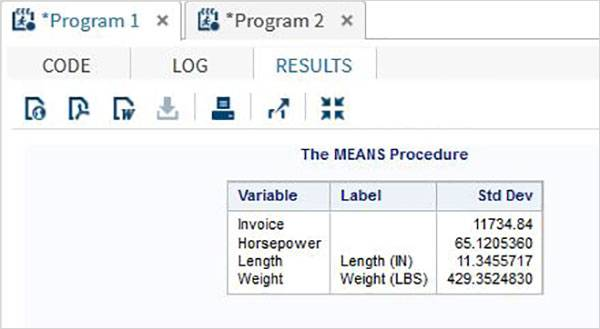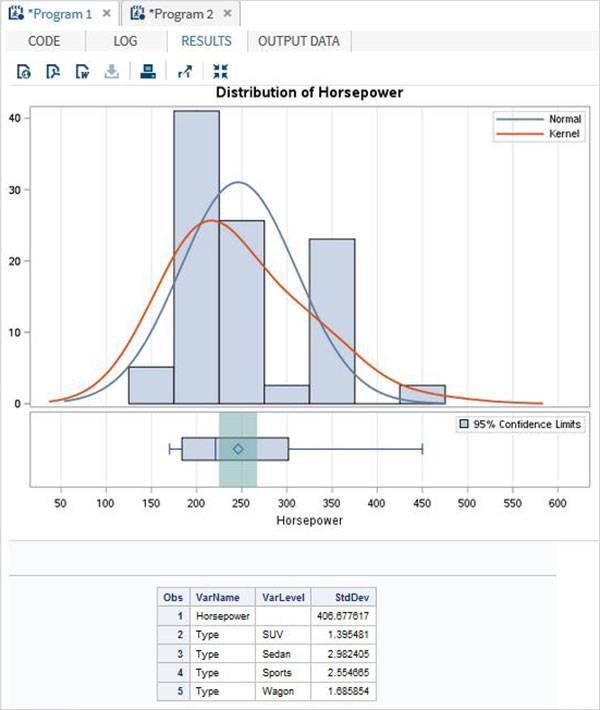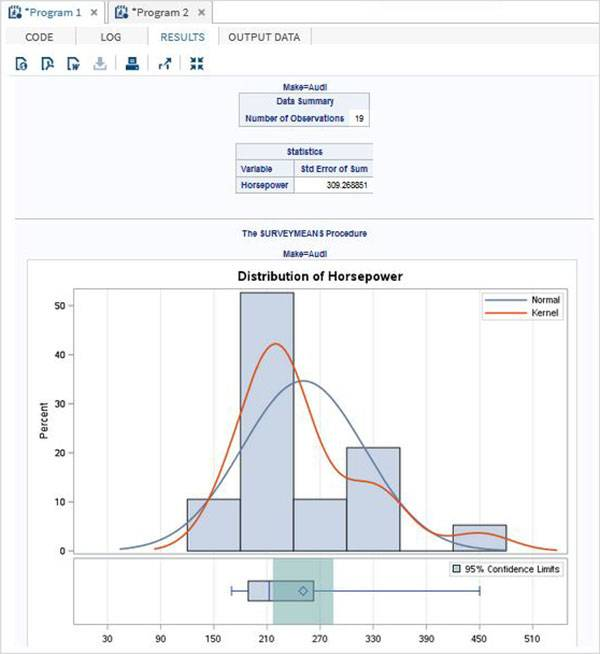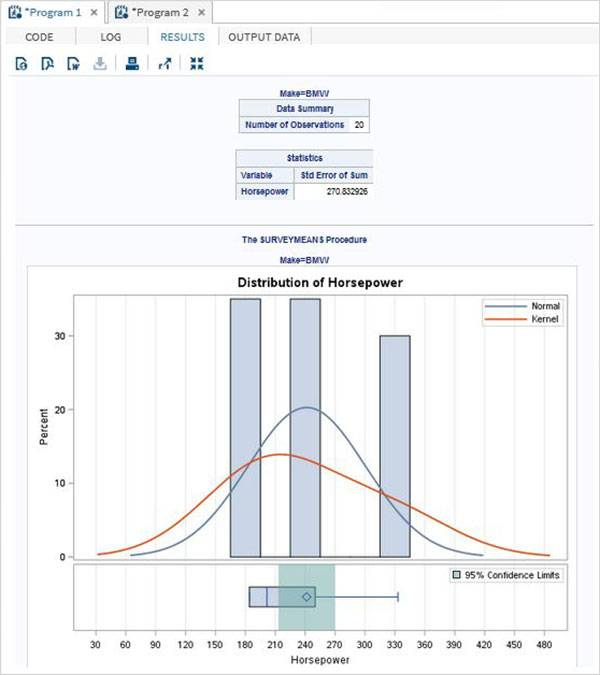# SAS标准偏差

## 语法

```PROC means DATA = dataset STD;
```

• Dataset是数据集的名称。

## 例

```PROC SQL;
create table CARS1 as
SELECT make,type,invoice,horsepower,length,weight
FROM
SASHELP.CARS
WHERE make in ('Audi','BMW')
;
RUN;

proc means data=CARS1 STD;
run;
```## 句法

```PROC SURVEYMEANS options statistic-keywords ;
BY variables ;
CLASS variables ;
VAR variables ;
```

• BY表示用于创建观察组的变量。
• CLASS表示用于分类变量的变量。
• VAR表示将计算SD的变量。

## 例

```proc surveymeans data=CARS1 STD;
class type;
var type horsepower;
ods output statistics=rectangle;
run;
proc print data=rectangle;
run;
```## 例

```proc surveymeans data=CARS1 STD;
var horsepower;
BY make;
ods output statistics=rectangle;
run;
proc print data=rectangle;
run;
```

### make =“Audi”的结果### make =“BMW”的结果## 您可能还喜欢：